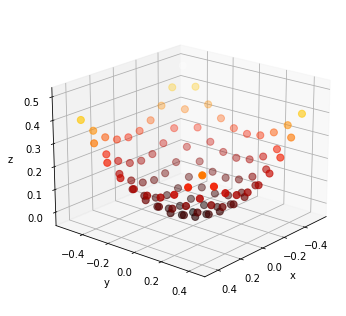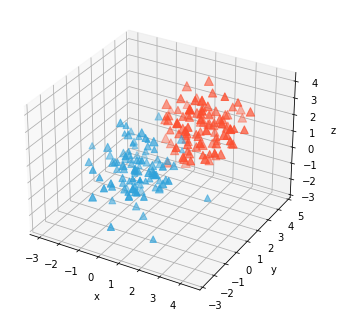# Scatter3D#

Title
Scatter3D Element
Dependencies
Matplotlib
Backends
Matplotlib
Plotly
```import numpy as np
import holoviews as hv
from holoviews import dim, opts

hv.extension('matplotlib')
````Scatter3D` represents three-dimensional coordinates which may be colormapped or scaled in size according to a value. They are therefore very similar to `Points` and `Scatter` types but have one additional coordinate dimension. Like other 3D elements the camera angle can be controlled using `azimuth`, `elevation` and `distance` plot options:

```y,x = np.mgrid[-5:5, -5:5] * 0.1
heights = np.sin(x**2+y**2)
hv.Scatter3D((x.flat, y.flat, heights.flat)).opts(
opts.Scatter3D(azimuth=40, elevation=20, color='z', s=50, cmap='fire'))
```Just like all regular 2D elements, `Scatter3D` types can be overlaid and will follow the default color cycle:

```(hv.Scatter3D(np.random.randn(100,4), vdims='Size') * hv.Scatter3D(np.random.randn(100,4)+2, vdims='Size')).opts(
opts.Scatter3D(s=(5+dim('Size'))*10, marker='^'))
```For full documentation and the available style and plot options, use `hv.help(hv.Scatter3D).`

This web page was generated from a Jupyter notebook and not all interactivity will work on this website. Right click to download and run locally for full Python-backed interactivity.